* indicates a required field• phone number format invalid• email format invalidProblems detected, please review the form.
Privacy - Terms

# News from Mathnasium of Lakewood CO

### Maps and Direct Paths

Aug 10, 2021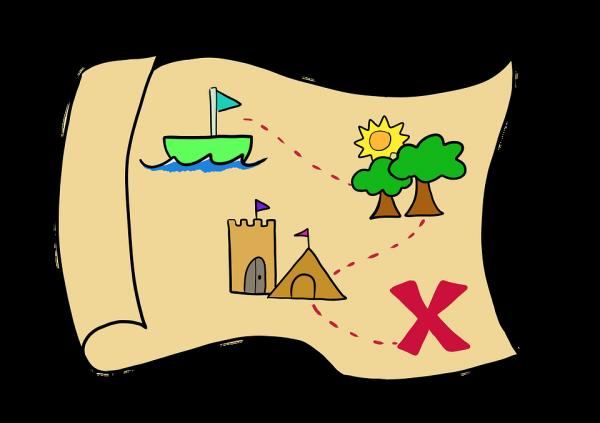Intro

When travelling, most people look for the easiest route or the shortest route from point A to point B. In some cases, we have multiple stops to make along the way that change our options/total distance travelled and, ultimately, how much time we spend traveling. This week we will be covering a Problem of the Week at the upper-elementary level that involves mapping a path and then determining relative positions between points (by counting boxes). We will then discuss a way to calculate the distance if flown overhead (the direct distance between two points — usually a diagonal). Let’s dive right in!

The Problem

Question: Chloe gets on her bike at home and rides 2 miles north to the post office. From there, she rides 3 miles west to the library. Then, she rides 4 miles south to the park, then 5 miles east to the farmer’s market. Finally, Chloe rides her bike 2 miles north to her friend Daniel’s house. How far away is Daniel’s house from Chloe’s house? In what direction?

First things first, let’s get some graph paper: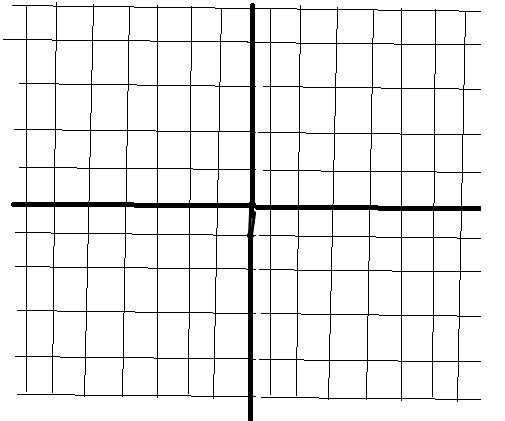There we go! Okay, we will choose north to be straight up, then east to the right, and so on: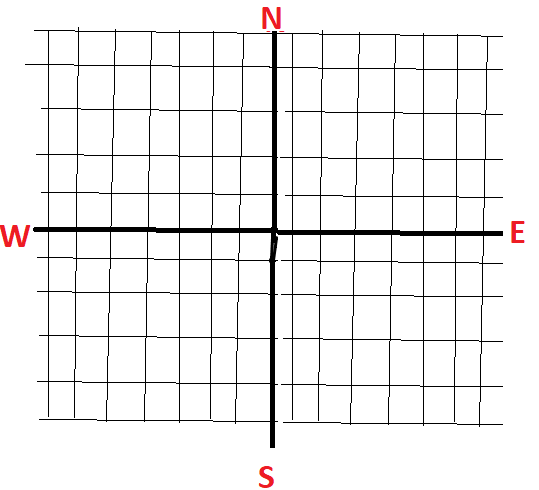Great! Now we choose a starting point. We start at Chloe’s house in the problem and we can choose any point on our graph to be the starting point (since all the distances are relative to Chloe’s house), so, to make it easy on ourselves, let’s choose the origin (0, 0) to be the starting point. This yields the following: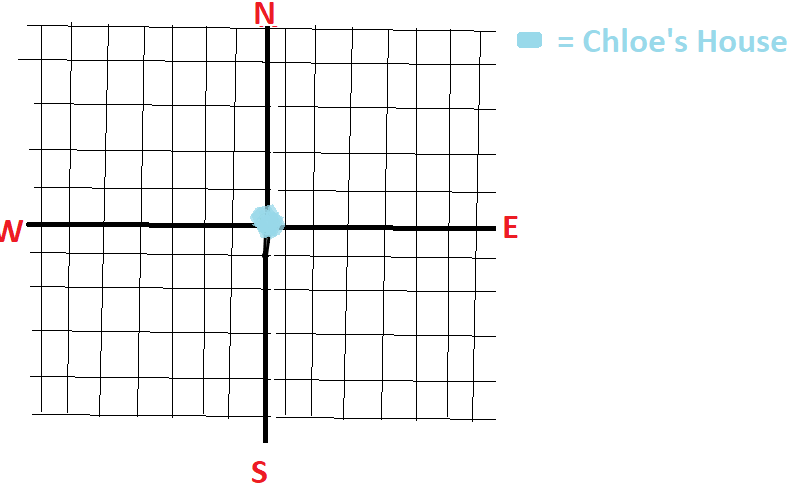Next we just follow the problem and ride the bike! Chloe rides 2 miles north to the post office, then 3 miles west to the library, then 4 miles south to the park, then 5 miles east to the farmers market, and finally, 2 miles north to Daniel’s house: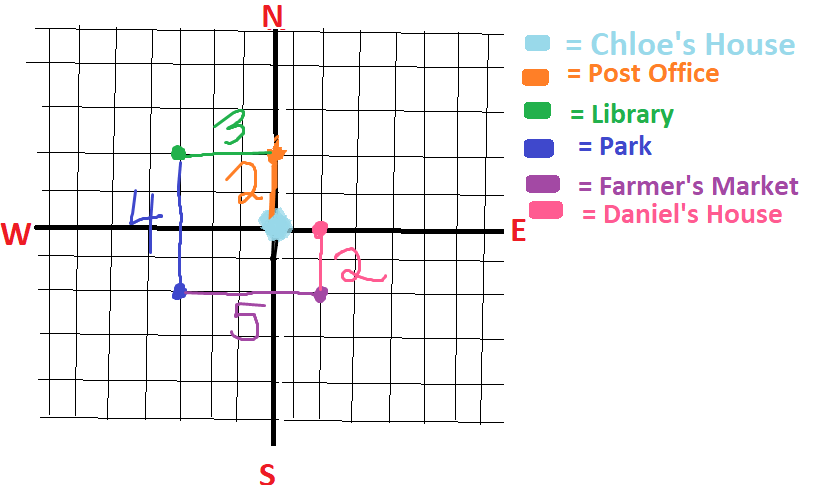Now we can just count the spaces from Chloe’s house (0, 0) to Daniel’s house (2, 0) and we find that Daniel’s house is 2 miles east of Chloe’s house! For many of these map type problems, it’s best to draw a picture as doing this mentally can prove to be a challenge if there are many steps (and/or dimensions — such as elevation or time). Next up we will be looking at the distance of the direct path between Chloe’s house and the park.

A Pythagorean Approach

When finding the distance of a line from one point directly to another it helps to build a right triangle and use the Pythagorean Theorem to find the length of the hypotenuse. Let’s take a look: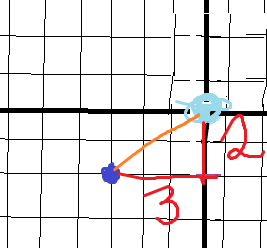In this picture, we connect the two points we are concerned about with an orange line, and we find the legs of the triangle it makes with the red lines. Our legs have lengths of 2 units and 3 units. Now let’s remember the Pythagorean Theorem: If a triangle is a right triangle with legs ‘a’ and ‘b’ then the length of the hypotenuse, c, can be determined by

a2 + b2 = c2.

So, we have our leg lengths (2 and 3) so let’s plug n’ chug:

22  = 4 and 32 = 9

4 + 9 = 13

So, c2 = 13

Thus, c =  √13 or approximately 3.6 units.

Note that we do not consider the negative option for the square root since distance cannot be negative!

We have done it: we have found the direct path from one point to another and we also have a consistent way to do so for the future!

Outro

Mapping our route can not only help us make more efficient decisions, but also help with our sense of direction and general knowledge of where we are and relative positions of other objects/places. As for finding the distance between two points — this is mostly used in optimization. If we have some statistic/unit we would like to minimize or maximize and it is measured as the distance between two functions/points, we can use the Pythagorean Theorem (and, intrinsically, the distance formula) to minimize/maximize that distance using calculus! Now you can use math to really minimize your traveling time! This is the gateway to optimization within math. Thanks Pythagoras!